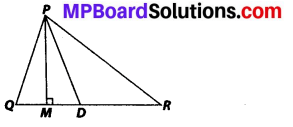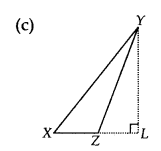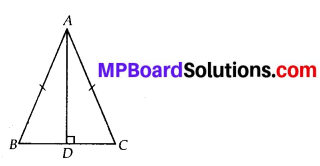# MP Board Class 7th Maths Solutions Chapter 6 The Triangles and Its Properties Ex 6.1

## MP Board Class 7th Maths Solutions Chapter 6 The Triangles and Its Properties Ex 6.1

Question 1.
In ∆PQR, D is the mid-point of $$\overline{Q R}$$.
$$\overline{P M}$$ is ___.
PD is ___.
Is QM = MR?Solution:
(i) PM is an altitude
(ii) PD is a median
(iii) No, QM ≠ MR.Question 2.
Draw rough sketches for the following:
(a) In ∆ABC, BE is a median.
(b) In ∆PQR, PQ and PR are altitudes of the triangle.
(c) In ∆XYZ, YL is an altitude in the exterior of the triangle.
Solution:Question 3.
Verify by drawing a diagram, if the median and altitude of an isosceles triangle can be same.
Solution:Draw a line segment AD perpendicular to BC. It can be observed that the length of BD and DC is also same. Therefore, AD is also a median of this triangle.